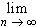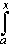#Interactive Real Analysis

Next | Previous | Glossary | Map

## 8.2. Uniform Convergence

### Theorem 8.2.11: Uniform Convergence and Differentiation

Let fn(x) be continuously differentiable functions defined on the interval [a, b]. If:
• the sequence fn converges pointwise to a function f, and
• the sequence of derivatives fn' converges uniformly
Then f is differentiable and
f'(x) =fn'(x)
for all x[a, b]. In other words, the limit process and the differentiation process can be switched in this case.

We already saw that neither pointwise nor uniform convergence by themselves were sufficient to switch limits and differentiation. But together things will work out, as we will see.

The sequence of derivatives fn' converges uniformly and each fn' is continuous. Thus the function defined as:

g(x) =fn'

is well defined and continuous. We also have by the fundamental theorem of calculus that:

fn(x) - fn(a) =fn' (x) dx

By a previous theorem we know that:fn' (t) dt =fn' (t) dt =g(t) dt

But then, together with the previous line, we get:fn(x) - fn(a) = f(x) - f(a) =g(t) dt
because the fn's converge pointwise to the function f. Thus:
f(x) =g(t) dt + f(a)

But the function on the right, being defined as an integral of a continuous function, is differentiable and therefore f must be differentiable. Indeed, we get that:

f'(x) = g(x) =fn'
which is what we wanted to prove.Next | Previous | Glossary | Map